# Acta Universitatis Danubius. Œconomica, Vol 13, No 3 (2017)

Œconomica

Cătălin Angelo Ioan1

Abstract: The paper establishes an equivalence of the Andrica’s conjecture in the direction of an increase of the difference of square root of primes by a combination of two consecutive primes.

Keywords: Andrica’s conjecture; prime

JEL Classification: C002

1. Introduction

In a previous paper, entitled “About Andrica’s conjecture” the authors have established an equivalence of conjecture Andrica by considering the ratio of two consecutive prime numbers. Because the average deviation calculated relative to the two terms, in this article will study another limit for the difference of square roots of two consecutive prime numbers.

A number pN, p2 is called prime number if its only positive divisors are 1 and p.

Even if do not know much about prime numbers, there exist a lot of attempts to determine some of their properties, many results being at the stage of conjectures.

A famous conjecture relative to prime numbers is that of Dorin Andrica. Denoting by pn - the n-th prime number (p1=2, p2=3, p3=5 etc.), Andrica’s conjecture () states that: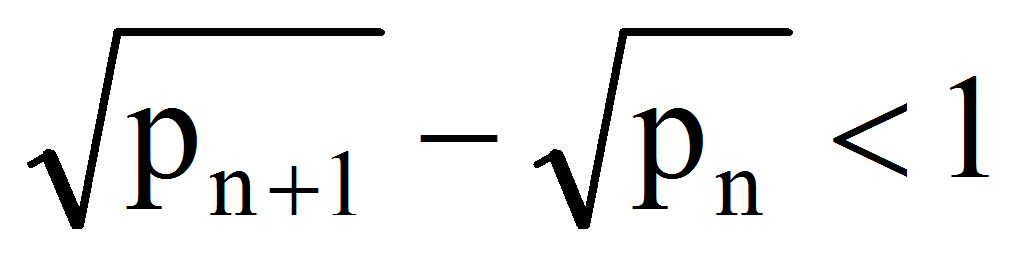n1

In  we have found the following:

Theorem

Let pn the n-th prime number. The following statements are equivalent for n5:

1.;

2. 0 such that: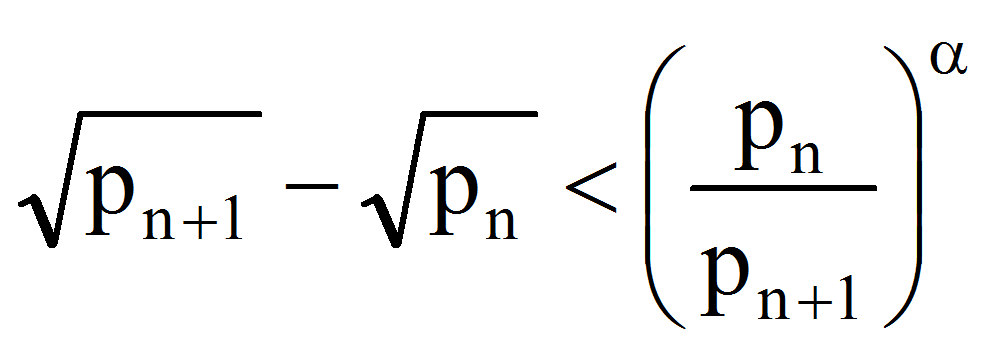.

In the following, we shall prove a stronger theorem of equivalence of Andrica’s conjecture.

2. Main Theorem

Theorem

Let pn the n-th prime number. The following statements are equivalent for n5:

1.;

2. 0 such that: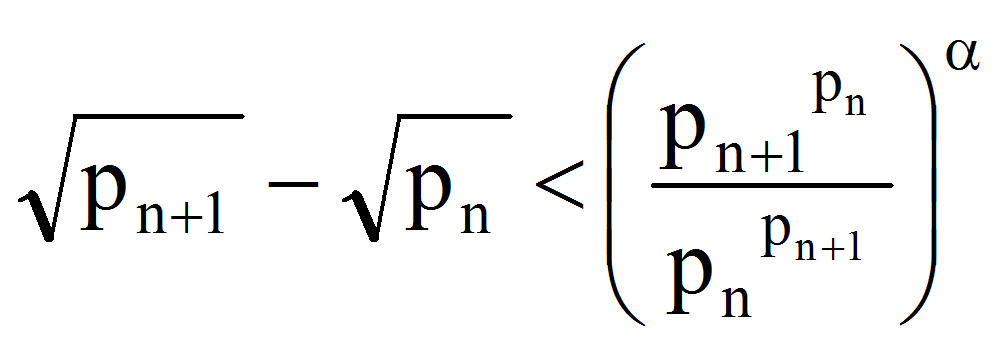.

Proof

First of all let the function f:[e,)R,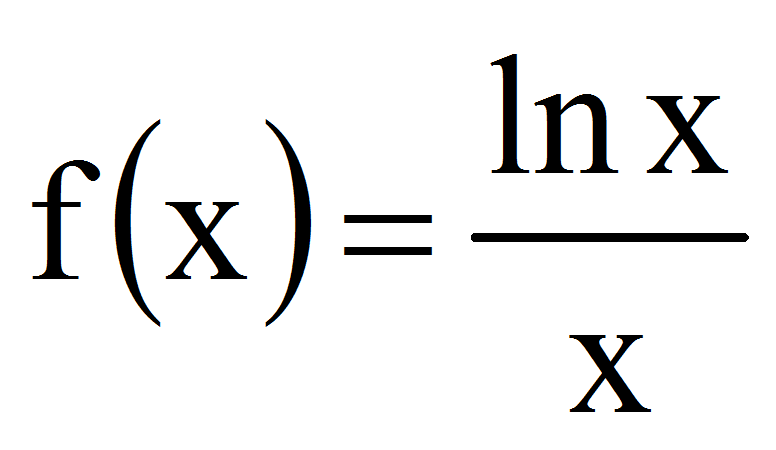. We have: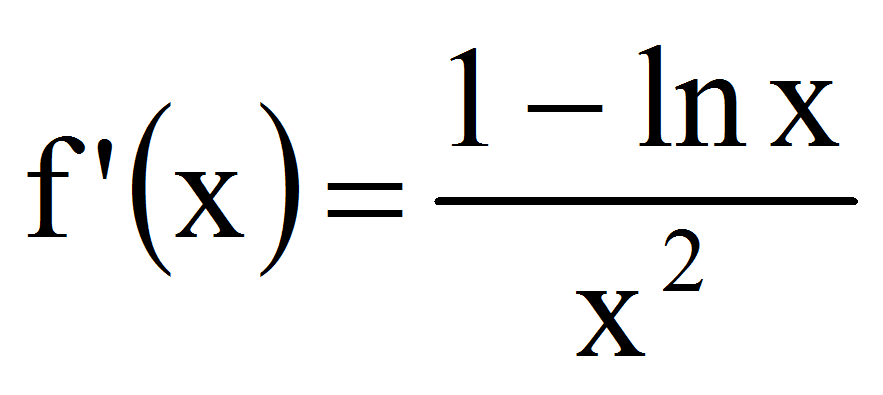0 therefore f is a strictly decreasing function. For n2 we have therefore: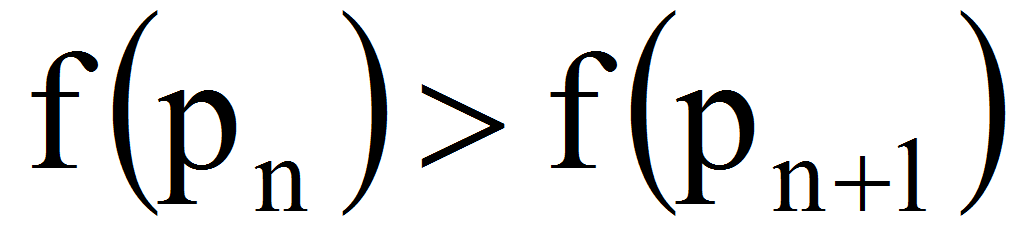that is: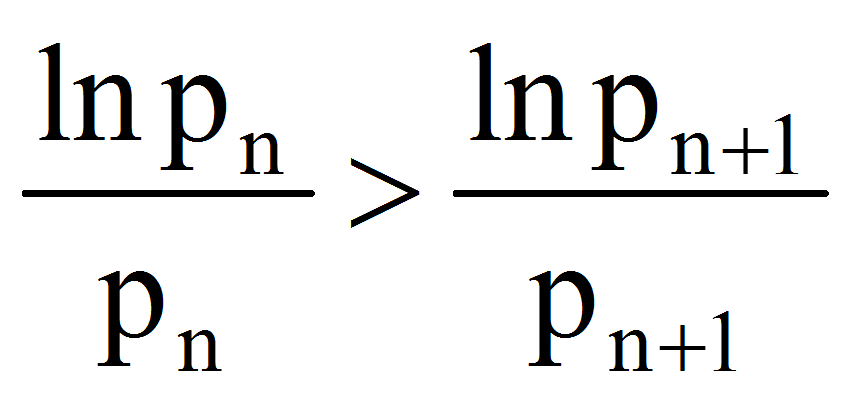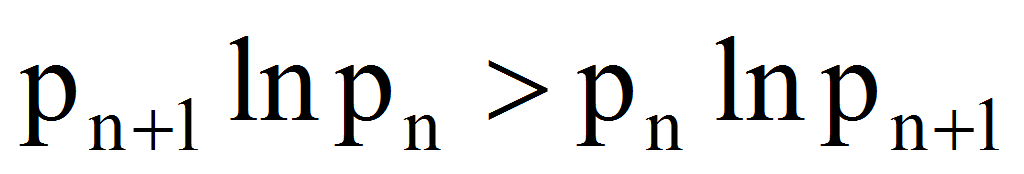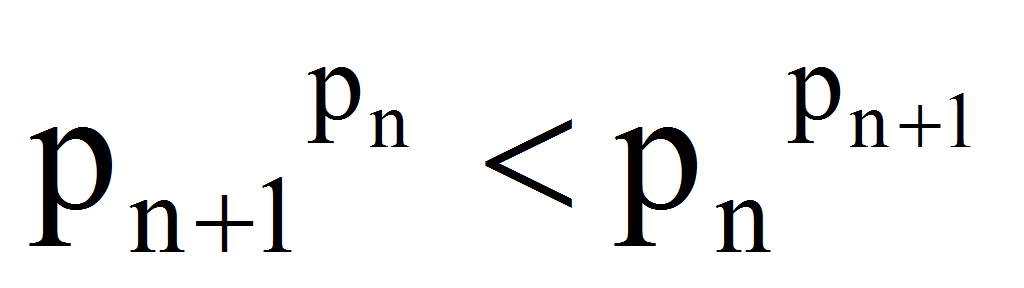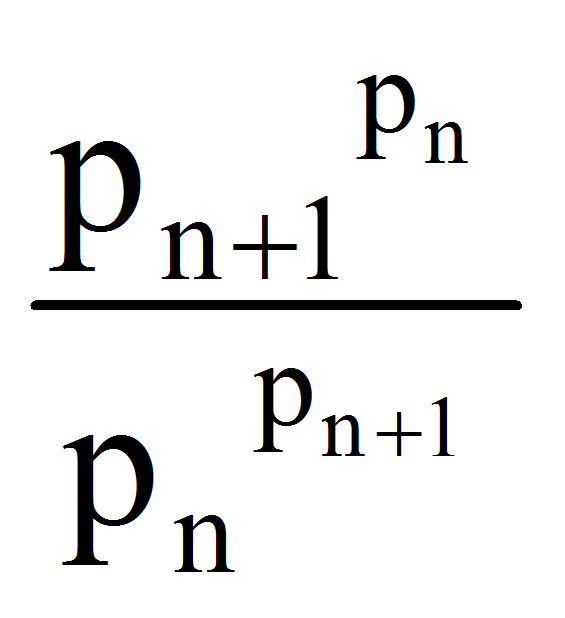1.

21 Because<1 follows that: 0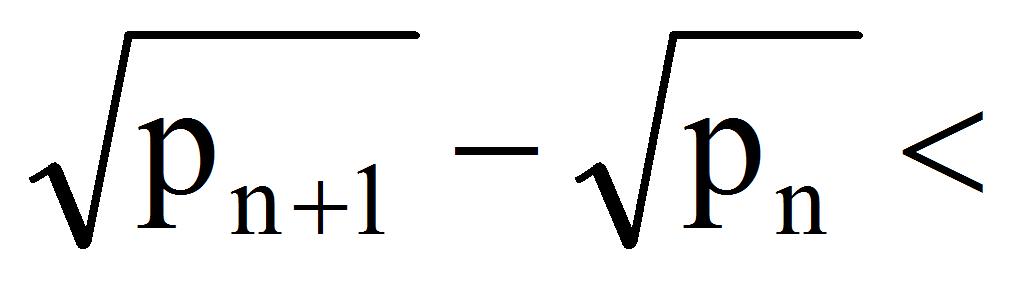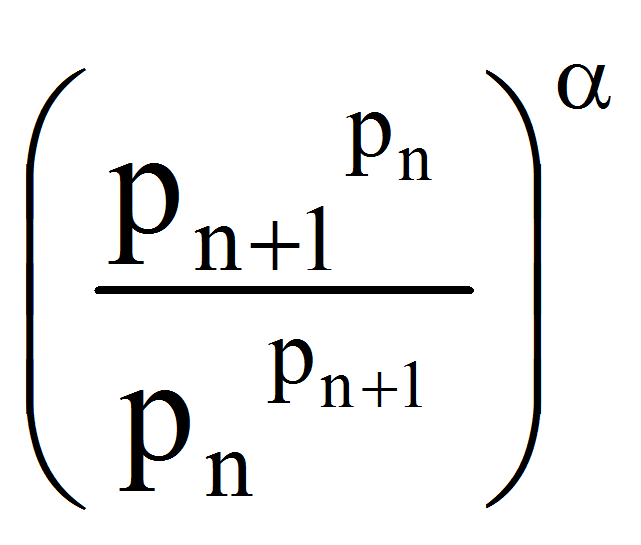<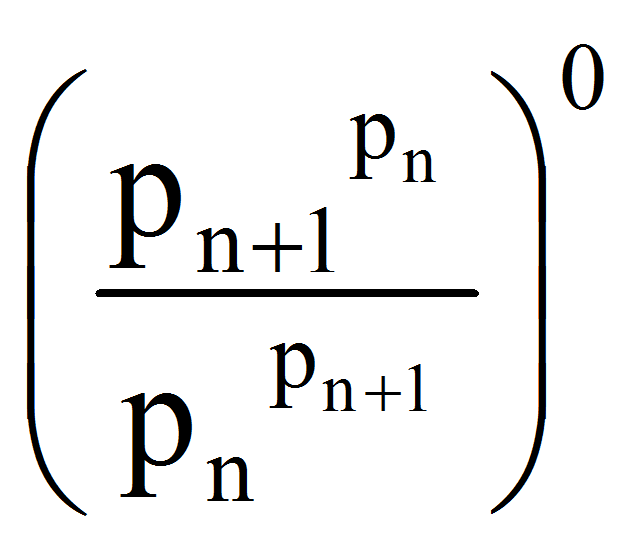=1.

12 If we take the logarithm in the relationship, it becomes: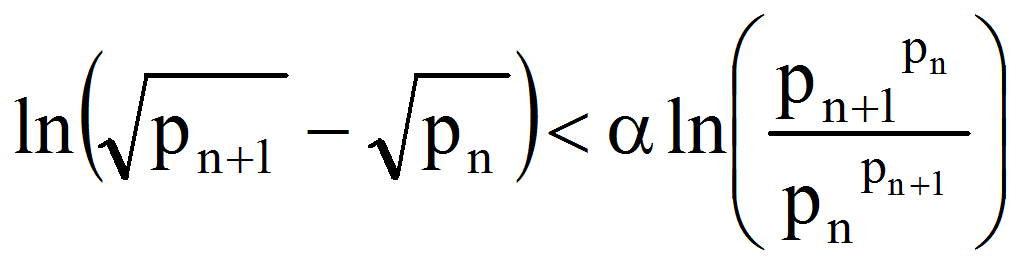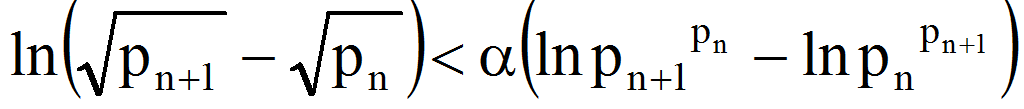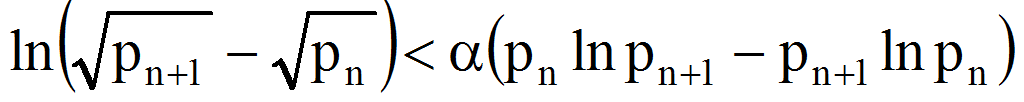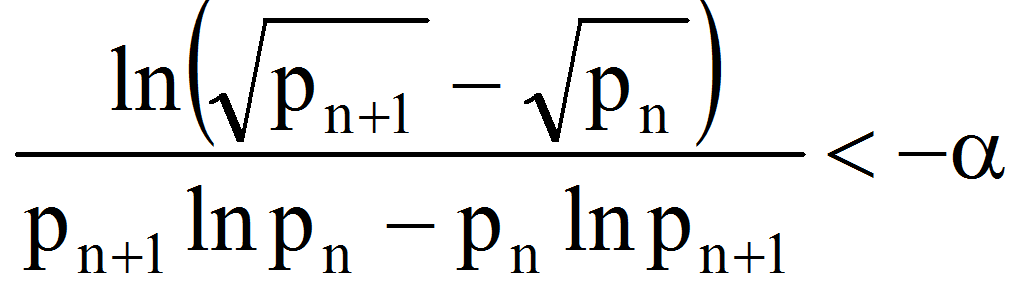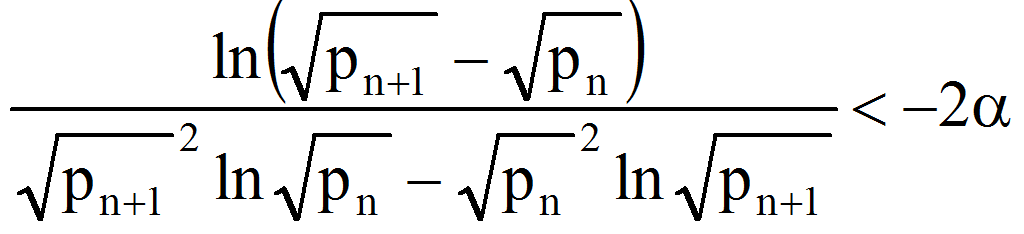.

Let now the function:

g:(a,)R,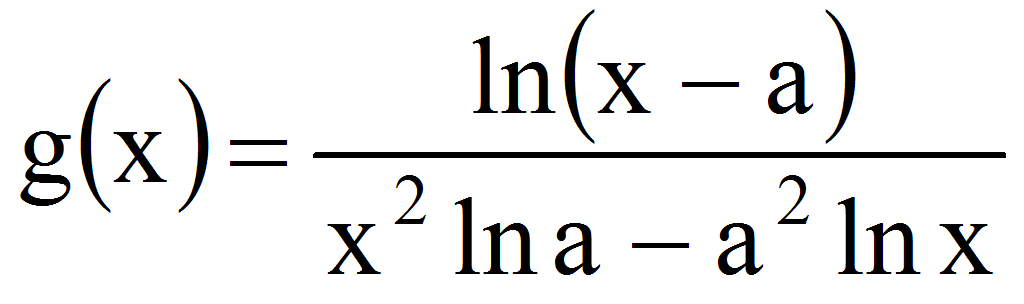with a>2

We have now: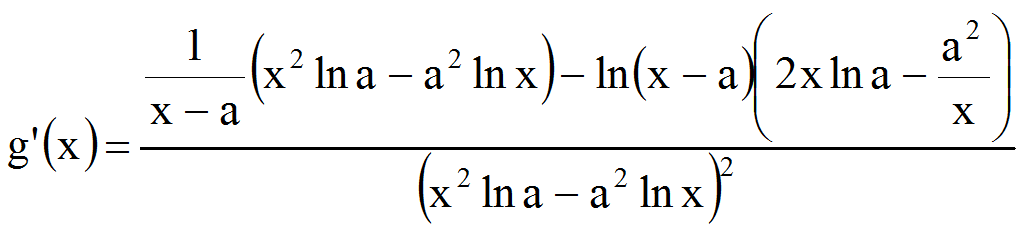=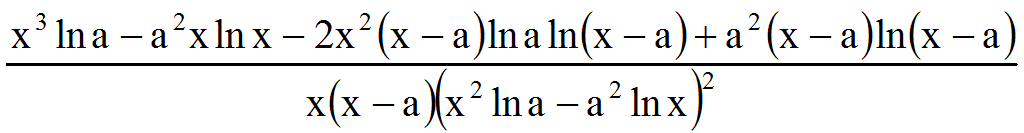Because the denominator of g’ is positive, we must inquire into the character of the function:

h:(a,)R,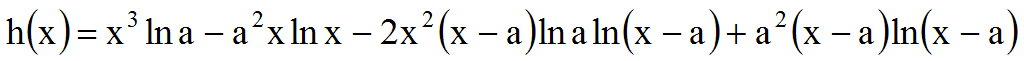.

Computing the derivative of h: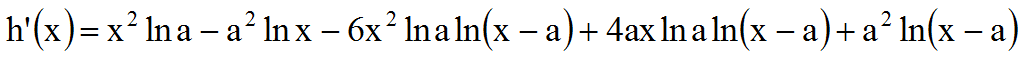Let now:

y:(a,)R,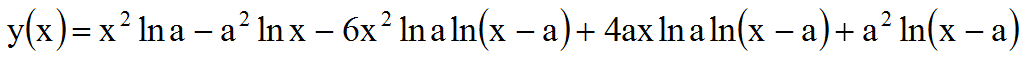and the derivative: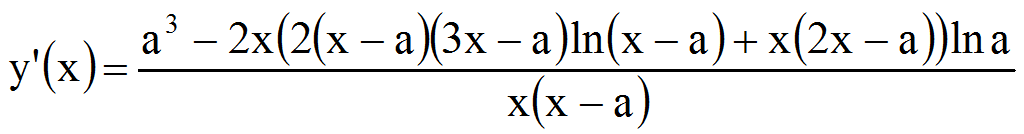Let now the function (the numerator of y):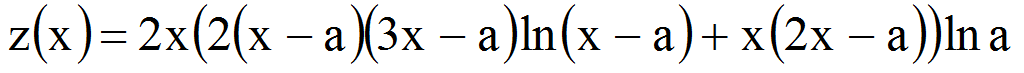and, also, the derivative: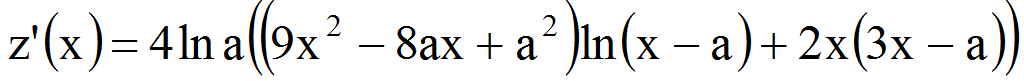Because xa we have that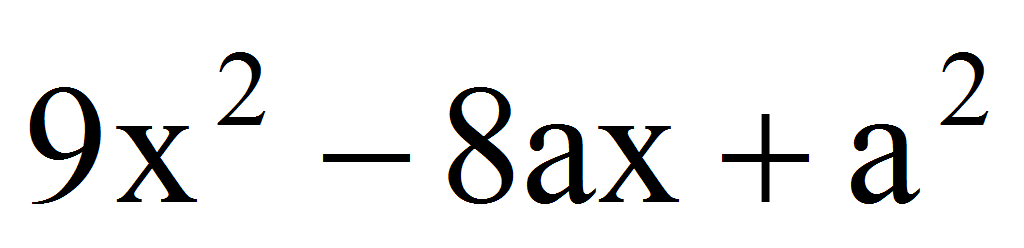0 therefore z is a strictly increasing function.

But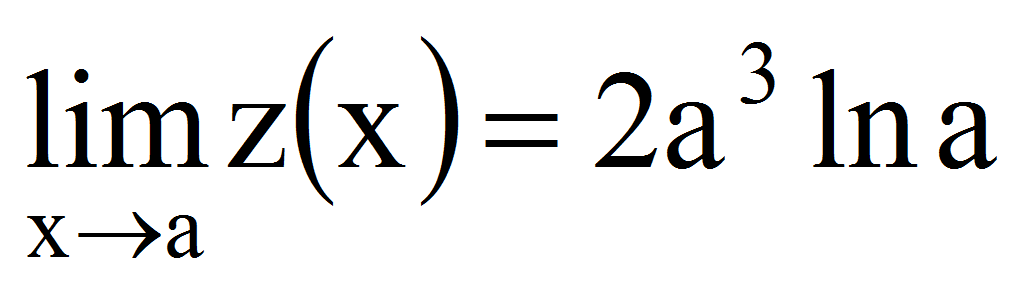0 therefore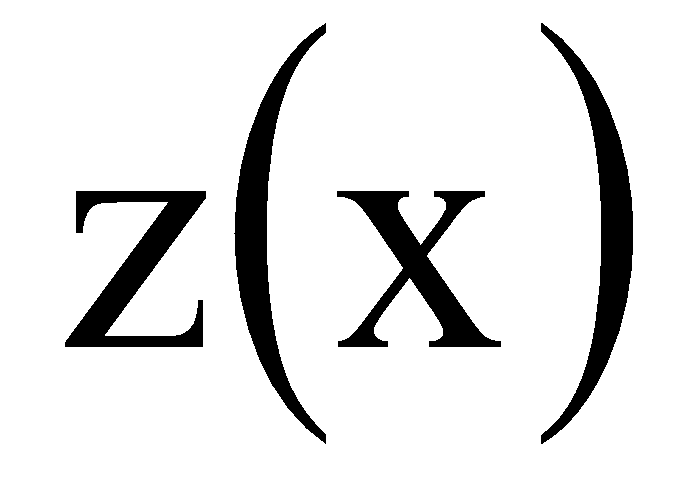0 xa.

In this case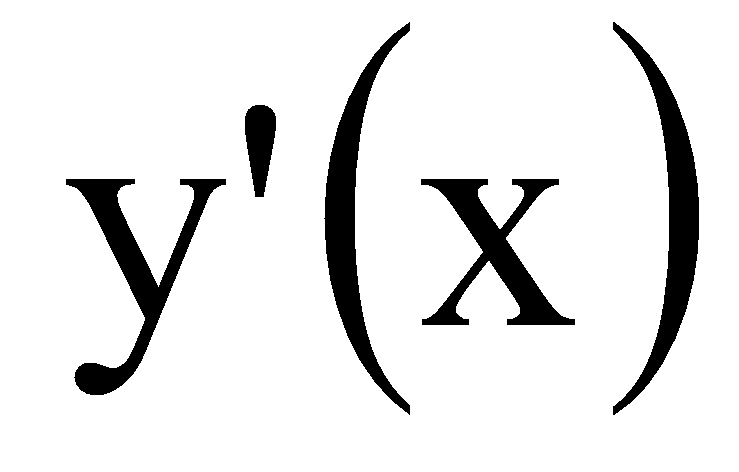0 then y is also a strictly increasing function.

But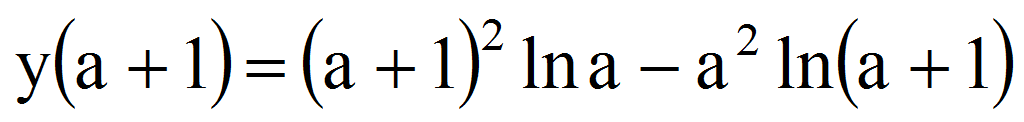=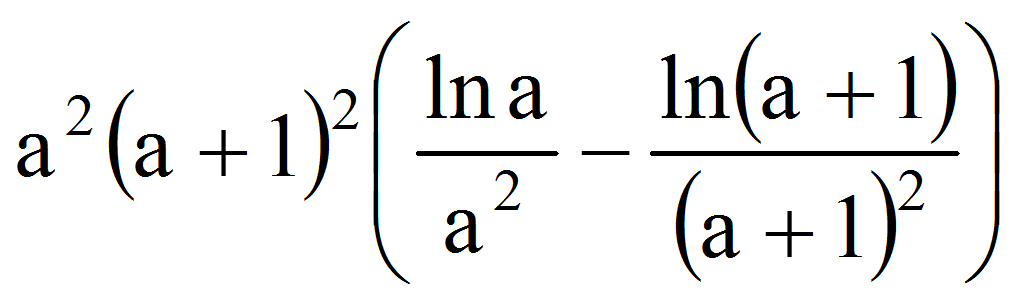.

The function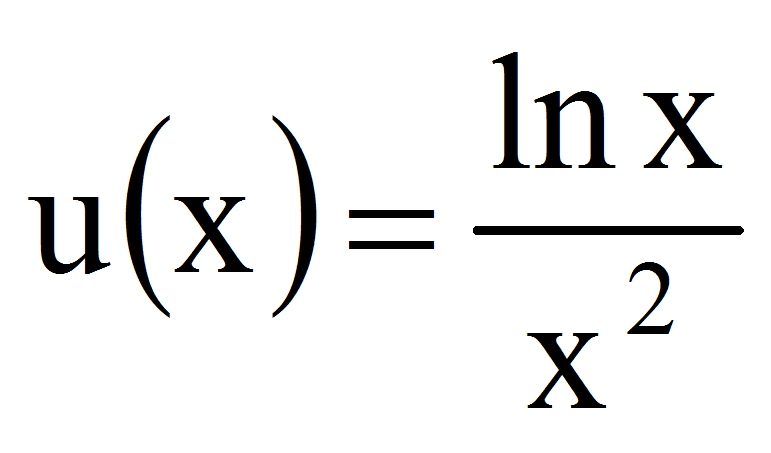has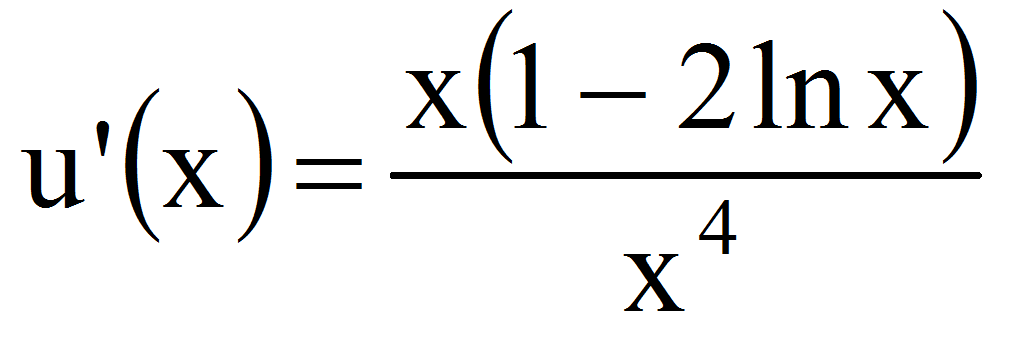0 for x2 therefore u is decreasing and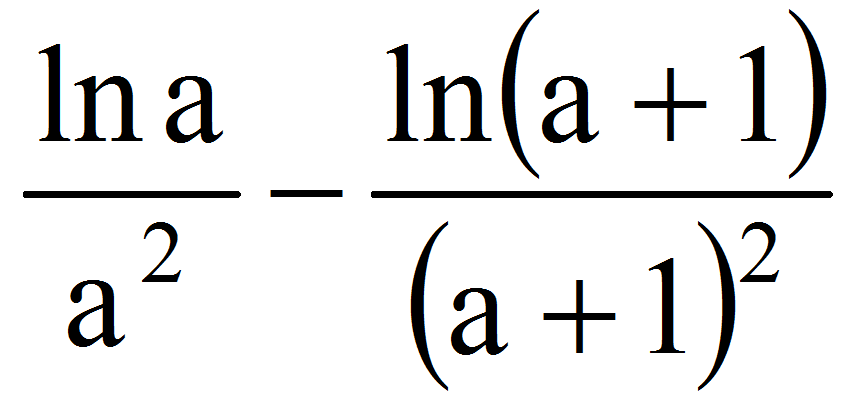=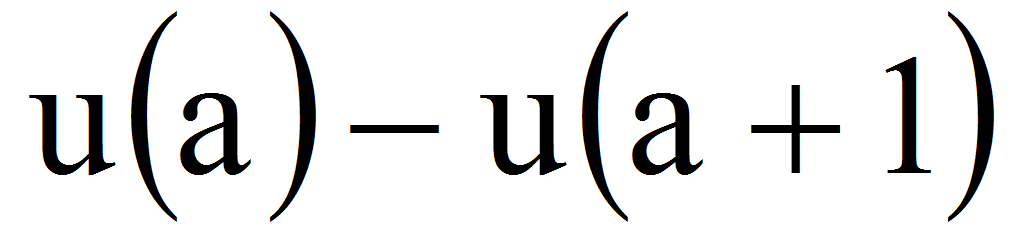0.

We have now: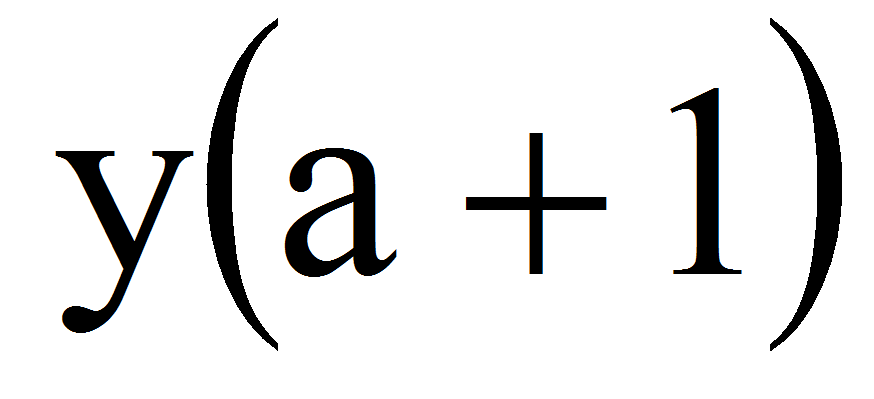0 therefore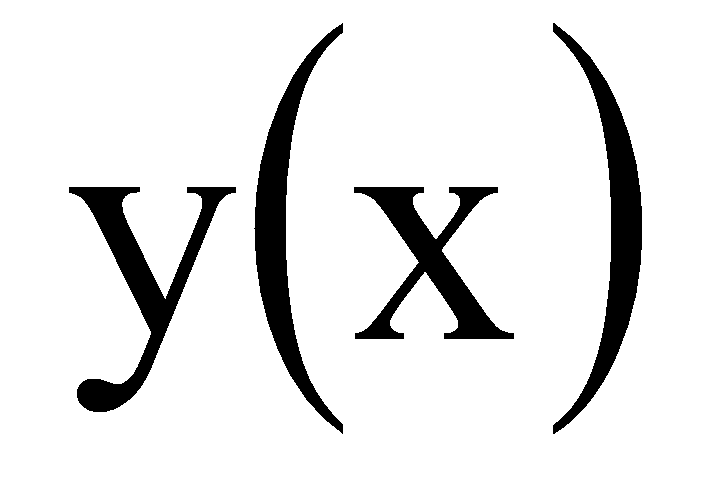0 for xa+1.

Now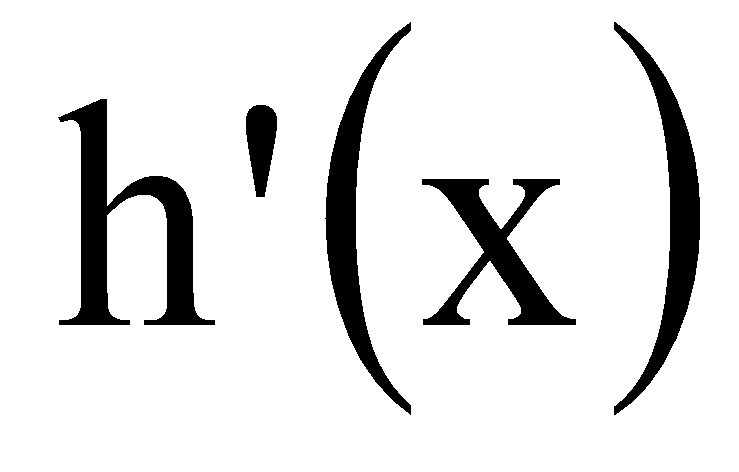0 which give us: h is increasing.

But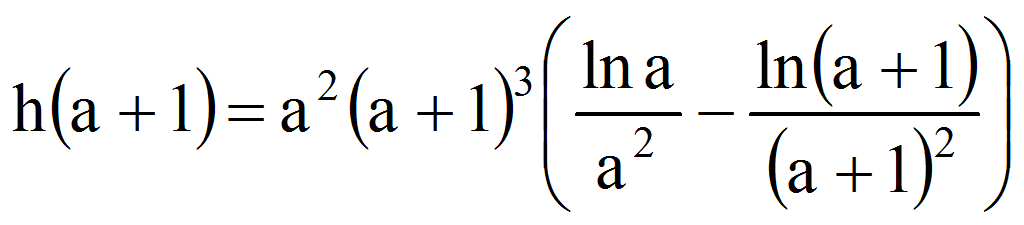0 therefore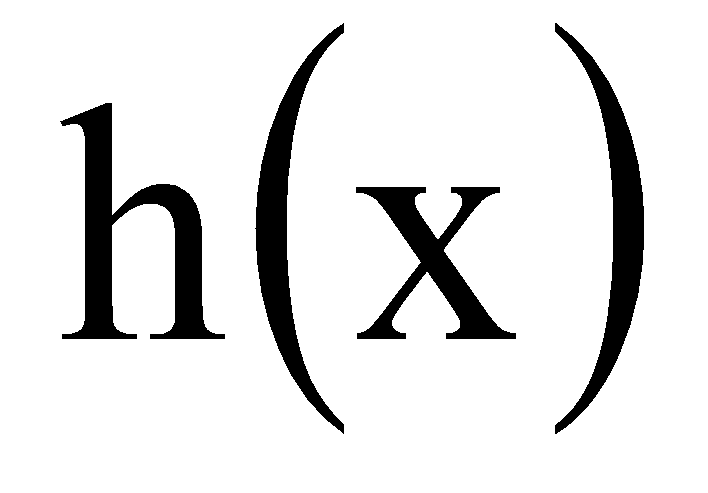0 for xa+1.

Because now: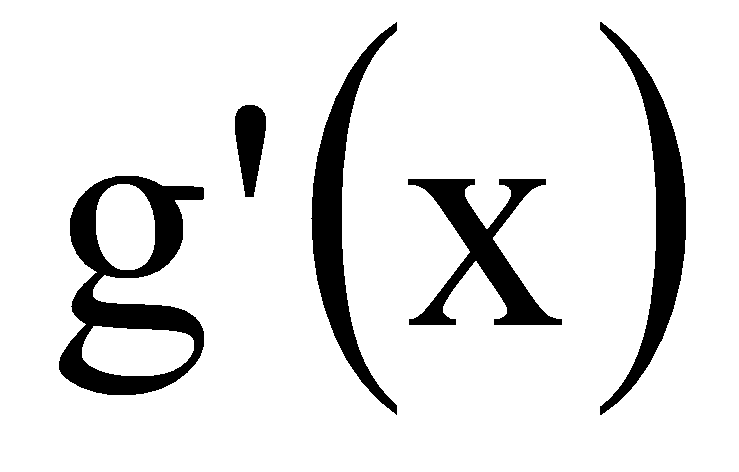0 implies that g is increasing and with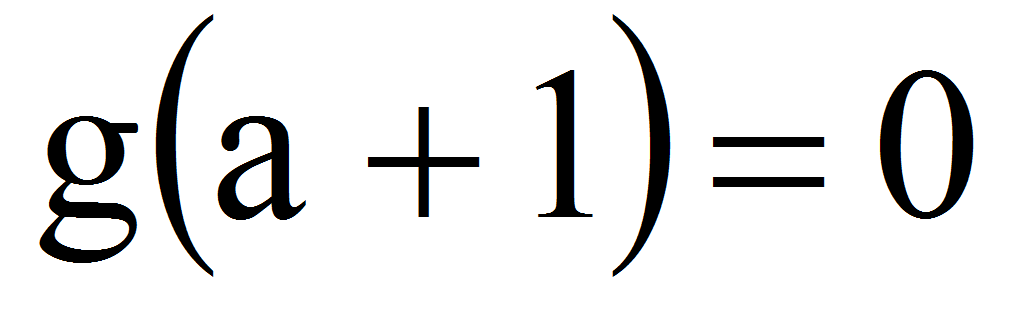we find that g(x)0 xa+1.

From hypothesis 1 (Andrica’s conjecture), we have: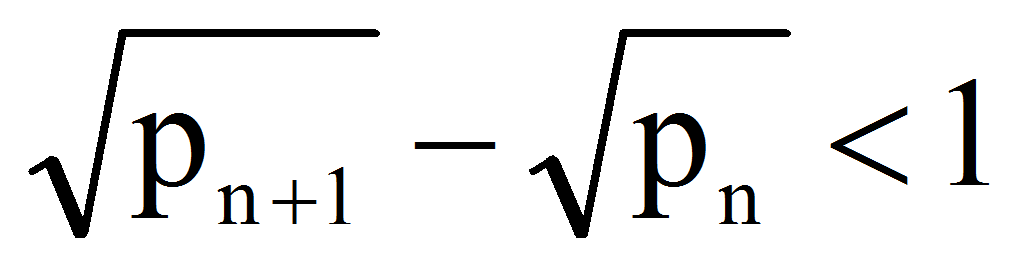and noting: x=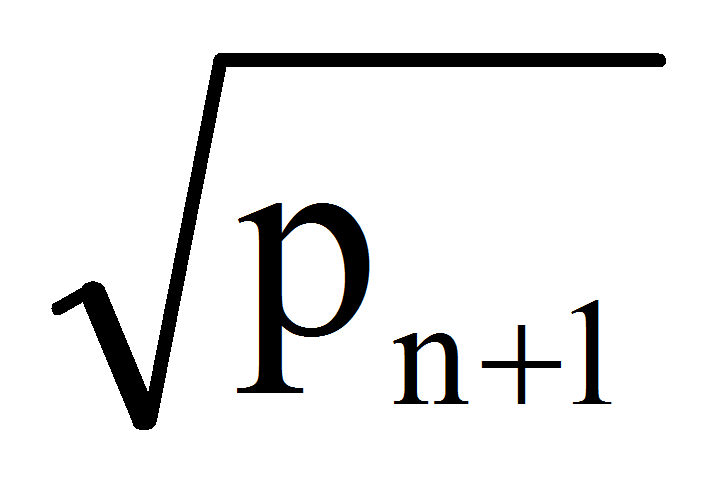, a=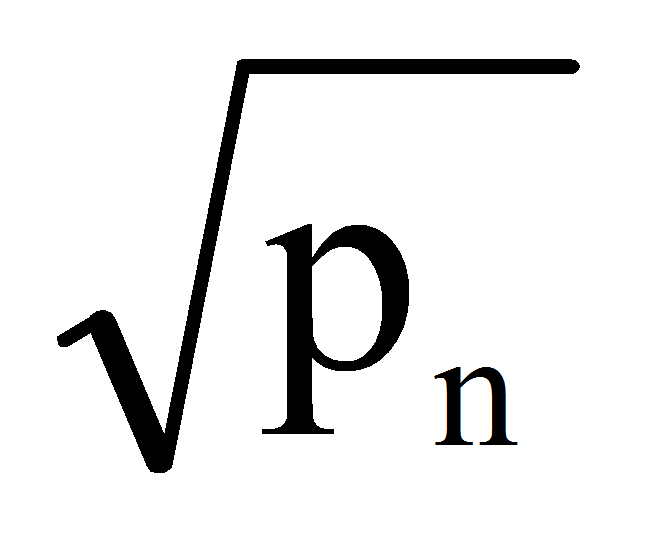we have: x(a,a+1) therefore: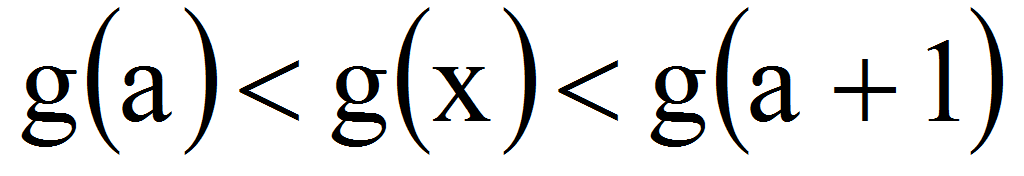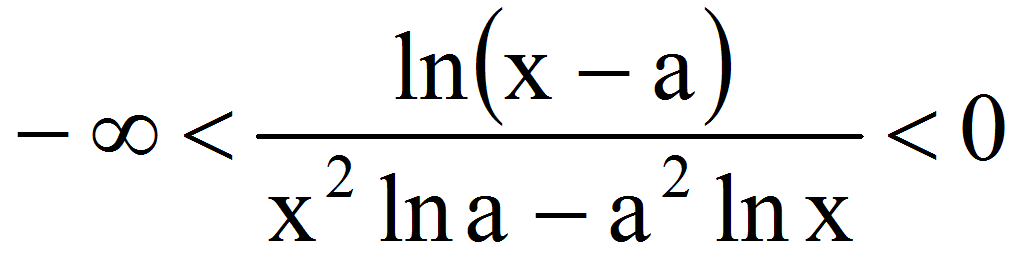Considering =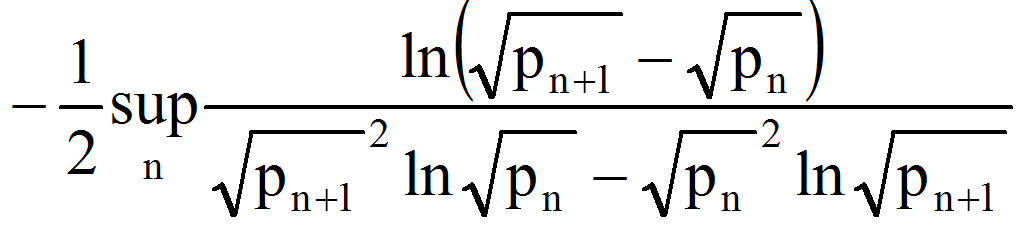0, the statement 2 is now obvious. Q.E.D.

3. Determination of the Constant

Using the Wolfram Mathematica software, in order to determine the constant (for the first 100000 prime numbers):

Clear[“Global`*”];

numberiterations=100000;

minimum=1000;

k=0;

For[i=5,i<=numberiterations+4, i++,

difference=Sqrt[Prime[i+1]]-Sqrt[Prime[i]];

ratio=Log[Prime[i+1]]*Prime[i]-Log[Prime[i]]*Prime[i+1];

log=Log[difference]/ratio;

If[log<minimum,minimum=log]];

Print["Minimum=",N[minimum,1000]]

we found that the first 1000 decimals are:

=0.001801787909180184090558881990879581852587815188626060829671181995518153228056185868661669722893637929905150138361741357987598217520912492958000134271102248291291440100211921382959611030962352046213123107738700539021075748371514085755924571808071605072827284127643779109598663531522374100243861797823777482028364380170936681469375189124611595038701054740899835310850858481264555165634252199160623380073272834451080219681979931918287609129486097360176969992548676629716572067527720901123119401797627368003734134881964963643241047796485654858914187103720570510400193723300037859727351479951565306627468352075884099806617621474175589423220844469527382500914548671086635285509959540990596065572675463044441151661992941475164500380927975560830750502367458528934167921925547374264915121574707114622773865335533852158934313781909407119398388818028233946073756228798804604974623153893100857242848052370682767307818661501668704604756723146711562022353266081970578858543065045549989697839196705824350226507331762.

4. References

Andrica, D. (1986). Note on a conjecture in prime number theory. Studia Univ. Babes-Bolyai Math. 31(4), pp. 44–48.

Guy, R.K. (1994). Unsolved Problems in Number Theory. Second Edition. Springer Verlag, New York.

Ioan, C.A. & Ioan, A.C. (2015). About Andrica’s conjecture. Acta Universitatis Danubius Œconomica, no 1, vol. 11, pp. 149-153.

Wolf, M. (2010). A Note on the Andrica Conjecture. arXiv:1010.3945v1 [math.NT] 19 Oct.

1 Associate Professor, PhD, Department of Economics, Danubius University of Galati, Romania, Address: 3 Galati Blvd., Galati 800654, Romania, Tel.: +40372361102, Corresponding author: catalin_angelo_ioan@univ-danubius.ro.

AUDŒ, Vol. 13, no. 3, pp. 207-212

10

### Refbacks

• There are currently no refbacks.